# Polyline

## Résumé

A Polyline object is a shape defined by one or more paths, in which a path is a series of connected segments.

## Discussion

Dans de nombreux workflows de géotraitement, vous devez éventuellement exécuter une opération spécifique à l'aide d'informations sur les coordonnées et la géométrie, sans vouloir nécessairement créer une nouvelle classe d'entités (temporaire), la remplir avec des curseurs, l'utiliser, puis la supprimer. Les objets géométrie peuvent alors être utilisés en entrée et en sortie afin de simplifier le géotraitement. Les objets géométrie peuvent être entièrement créés à l'aide des classes Geometry, Multipoint, PointGeometry, Polygon ou Polyline.

During the creation of a geometry object, a simplification process is performed to make the geometry topologically consistent according to its geometry type. For instance, it rectifies polygons that may be self-intersecting, or contain incorrect ring orientations. Coordinates may move within the resolution of the geometry's spatial reference.

The following numeric operators can be used in place of geometry methods:

Geometry methodNumeric operator

intersect

+

union

|

difference

-

symmetricDifference

^

For example, to union two geometry objects, g3 = g1 | g2 is equivalent to g3 = g1.union(g2).

The + operator will use the geometry type (dimension) of the first geometry to determine the geometry type of the output.

## Syntaxe

` Polyline  (inputs, {spatial_reference}, {has_z}, {has_m})`
 Paramètre Explication Type de données inputs The coordinates used to create the object. The data type can be either Point or Array objects. Object spatial_reference The spatial reference of the new geometry.(La valeur par défaut est None) SpatialReference has_z The Z state: True for geometry if Z is enabled and False if it is not. (La valeur par défaut est False) Boolean has_m The M state: True for geometry if M is enabled and False if it is not.(La valeur par défaut est False) Boolean

## Propriétés

PropriétéExplicationType de données
JSON
(Lecture seule)

Returns an Esri JSON representation of the geometry as a string.

##### Conseil :

The returned string can be converted to a dictionary using the json module's loads function.

String
WKB
(Lecture seule)

Returns the well-known binary (WKB) representation for OGC geometry. It provides a portable representation of a geometry value as a contiguous stream of bytes.

Bytearray
WKT
(Lecture seule)

Returns the well-known text (WKT) representation for OGC geometry. It provides a portable representation of a geometry value as a text string.

Any true curves in the geometry will be densified into approximate curves in the WKT string.

String
area
(Lecture seule)

The area of a polygon feature. It is zero for all other feature types.

Double
centroid
(Lecture seule)

The true centroid if it is within or on the feature; otherwise, the label point is returned.

Point
extent
(Lecture et écriture)

The extent of the geometry.

Extent
firstPoint
(Lecture seule)

The first coordinate point of the geometry.

Point
hasCurves
(Lecture seule)

Returns True if the geometry has a curve.

Boolean
hullRectangle
(Lecture seule)

A space-delimited string of the coordinate pairs of the convex hull rectangle.

String
isMultipart
(Lecture seule)

Returns True if the number of parts for this geometry is more than one.

Boolean
labelPoint
(Lecture seule)

The point at which the label is located. The labelPoint is always located within or on a feature.

Point
lastPoint
(Lecture seule)

The last coordinate of the feature.

Point
length
(Lecture seule)

The length of the linear feature. The calculation uses 2D Cartesian mathematics.

For point and multipoint geometry, the length will be zero. For polygon geometry, the length will be the 2D length of the boundary.

Double
length3D
(Lecture seule)

The 3D length of the linear feature. The calculation uses 3D Cartesian mathematics.

For point and multipoint geometry, the length will be zero. For polygon geometry, the length will be the 3D length of the boundary.

##### Attention :

The operation does not perform unit conversion between the horizontal and the vertical coordinate systems. The x, y, and z coordinates are assumed to be in the same linear unit.

##### Attention :

This property is only available for projected data.

Double
partCount
(Lecture seule)

The number of geometry parts for the feature.

Integer
pointCount
(Lecture seule)

The total number of points for the feature.

Integer
spatialReference
(Lecture seule)

The spatial reference of the geometry.

SpatialReference
trueCentroid
(Lecture seule)

The center of gravity for a feature.

Point
type
(Lecture seule)

The geometry type: polygon, polyline, point, multipoint, multipatch, dimension, or annotation.

String

## Vue d’ensemble des méthodes

 Méthode Explication boundary () Constructs the boundary of the geometry.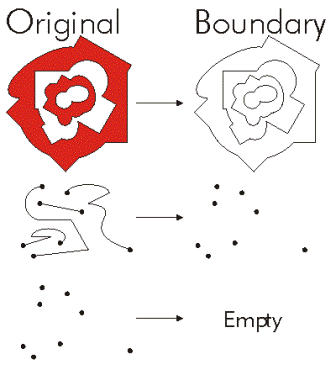buffer (distance) Constructs a polygon at a specified distance from the geometry.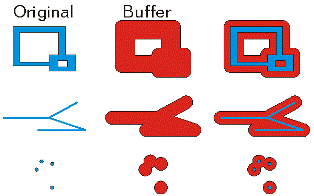clip (envelope) Constructs the intersection of the geometry and the specified extent.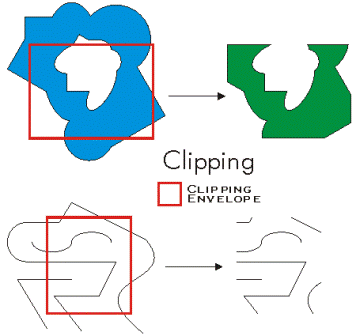contains (second_geometry, {relation}) Indicates if the base geometry contains the comparison geometry. contains is the opposite of within. Only True relationships are shown in this illustration.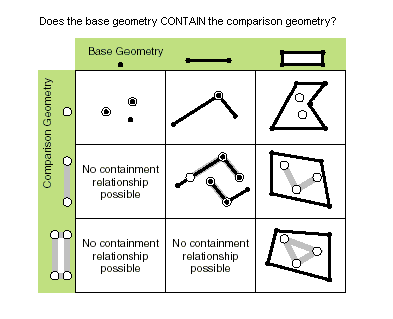convexHull () Constructs the geometry that is the minimal bounding polygon such that all outer angles are convex.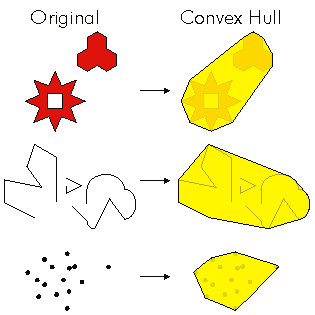crosses (second_geometry) Indique si les deux géométries s'intersectent dans une géométrie de type de forme inférieure. Deux polylignes se croisent si elles partagent uniquement des points, dont au moins un n'est pas une extrémité. Une polyligne et un polygone se croisent s'ils partagent une polyligne ou un point (pour une ligne verticale) à l'intérieur du polygone qui n'est pas équivalent à la polyligne entière. Only True relationships are shown in this illustration.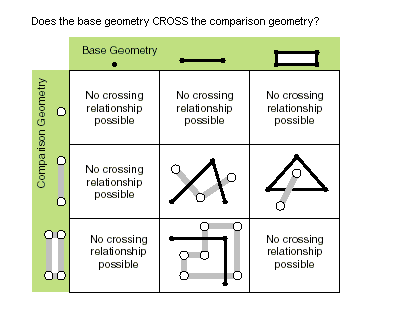cut (cutter) Splits this geometry into a part left of the cutting polyline, and a part right of it. When a polyline or polygon is cut, it is split where it intersects the cutter polyline. Each piece is classified as left of or right of the cutter. This classification is based on the orientation of the cutter line. Parts of the target polyline that do not intersect the cutting polyline are returned as part of the right of result for that input polyline. If a geometry is not cut, the left geometry will be empty (None).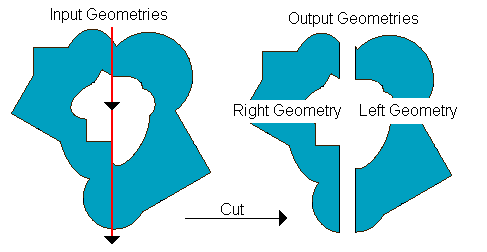densify (type, distance, {deviation}) Creates a new geometry with added vertices. difference (other) Constructs the geometry that is composed only of the region unique to the base geometry but not part of the other geometry. The following illustration shows the results when the red polygon is the source geometry.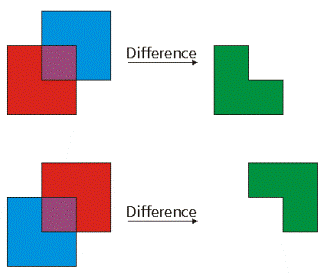disjoint (second_geometry) Indique si la géométrie de base et la géométrie de comparaison n'ont aucun point en commun. Deux géométries s'intersectent si la propriété disjoint renvoie False. Only True relationships are shown in this illustration.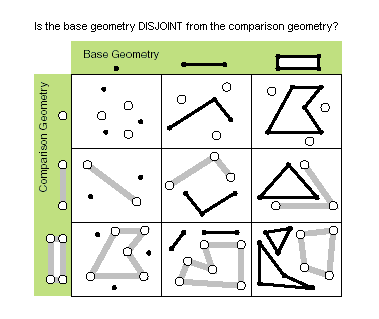distanceTo (other) Returns the minimum distance between two geometries. The distance is in the units of the geometry's spatial reference. If the geometries intersect, the minimum distance is 0.Both geometries must have the same projection. equals (second_geometry) Indicates if the base and comparison geometries are of the same shape type and define the same set of points in the plane. This is a 2D comparison only; M and Z values are ignored.Only True relationships are shown in this illustration.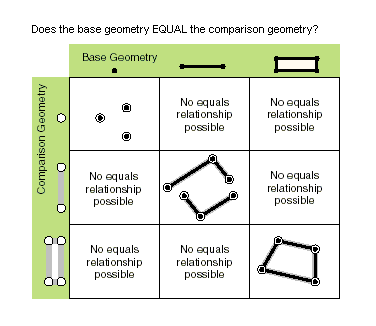generalize (max_offset) Creates a new simplified geometry using a specified maximum offset tolerance. getLength ({measurement_type}, {units}) Returns the length of the feature using a measurement type. getPart ({index}) Returns an Array object of Point objects for a particular part of the geometry if an index is specified. If an index is not specified, an Array object containing an Array of Point objects for each geometry part is returned. The getPart method is equivalent to indexing an object; that is, obj.getPart(0) is equivalent to obj. intersect (other, dimension) Constructs a geometry that is the geometric intersection of the two input geometries. Different dimension values can be used to create different shape types. The intersection of two geometries of the same shape type is a geometry containing only the regions of overlap between the original geometries.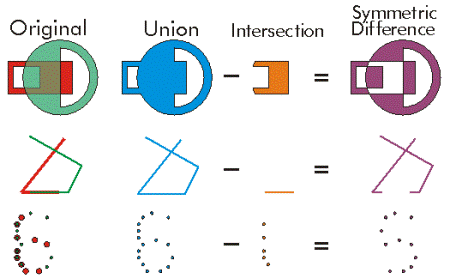For faster results, test if the two geometries are disjoint before calling intersect. measureOnLine (in_point, {use_percentage}) Returns a measure from the start point of this line to the in_point. overlaps (second_geometry) Indique si l'intersection des deux géométries possède le même type de forme que l'une des géométries en entrée et qu'elle n'est pas équivalente à l'une des géométries en entrée. Only True relationships are shown in this illustration.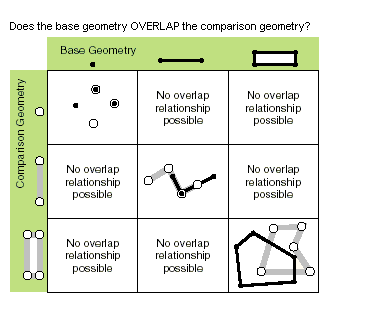pointFromAngleAndDistance (angle, distance, {method}) Returns a point at a given angle in degrees and distance in the units of the geometry's spatial reference using the specified measurement type. positionAlongLine (value, {use_percentage}) Returns a point on a line at a specified distance from the beginning of the line. projectAs (spatial_reference, {transformation_name}) Projects a geometry and optionally applies a geotransformation. To project, the geometry must have a spatial reference, and not have an unknown coordinate system. The new spatial reference system passed to the method defines the output coordinate system. If either spatial reference is unknown, the coordinates will not be changed. The z- and m-values are not changed by the projectAs method. queryPointAndDistance (in_point, {as_percentage}) Finds the point on the polyline nearest to the in_point and the distance between those points. Also returns information about the side of the line the in_point is on as well as the distance along the line where the nearest point occurs. segmentAlongLine (start_measure, end_measure, {use_percentage}) Returns a Polyline between start and end measures. Similar to Polyline.positionAlongLine but will return a polyline segment between two points on the polyline instead of a single point. snapToLine (in_point) Returns a new point based on in_point snapped to this geometry. symmetricDifference (other) Constructs the geometry that is the union of two geometries minus the instersection of those geometries.The two input geometries must be the same shape type.touches (second_geometry) Indicates if the boundaries of the geometries intersect. Two geometries touch when the intersection of the geometries is not empty, but the intersection of their interiors is empty. For example, a point touches a polyline only if the point is coincident with one of the polyline end points. Only True relationships are shown in this illustration.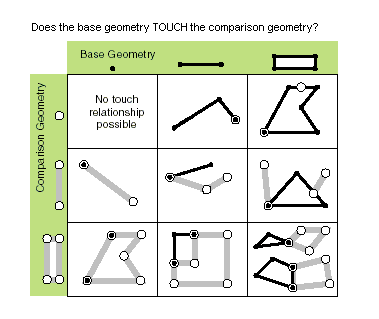union (other) Constructs the geometry that is the set-theoretic union of the input geometries. The two geometries being unioned must be the same shape type.within (second_geometry, {relation}) Indicates if the base geometry is within the comparison geometry. within is the opposite operator of contains. Only True relationships are shown in this illustration.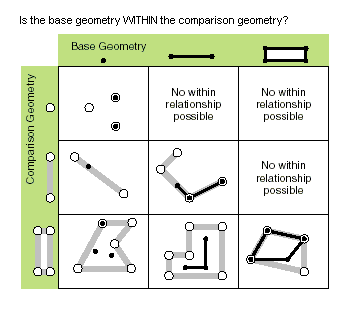The base geometry is within the comparison geometry if the base geometry is the intersection of the geometries and the intersection of their interiors is not empty. within is a Clementini operator, except in the case of an empty base geometry.

## Méthodes

`boundary ()`
Valeur renvoyée
 Type de données Explication Object A polygon's boundary is a polyline. A polyline's boundary is a multipoint, corresponding to the endpoints of the line. A point or multipoint's boundary is an empty point or multipoint.
`buffer (distance)`
 Paramètre Explication Type de données distance The buffer distance.The buffer distance is in the same units as the geometry that is being buffered. A negative distance can only be specified against a polygon geometry. Double
Valeur renvoyée
 Type de données Explication Polygon The buffered polygon geometry.
`clip (envelope)`
 Paramètre Explication Type de données envelope An Extent object used to define the clip extent. Extent
Valeur renvoyée
 Type de données Explication Object An output geometry clipped to the specified extent.
`contains (second_geometry, {relation})`
 Paramètre Explication Type de données second_geometry A second geometry. Object relation The spatial relationship type.BOUNDARY— Relationship has no restrictions for interiors or boundaries.CLEMENTINI— Interiors of geometries must intersect. Specifying CLEMENTINI is equivalent to specifying None. This is the default.PROPER— Boundaries of geometries must not intersect.(La valeur par défaut est None) String
Valeur renvoyée
 Type de données Explication Boolean A return Boolean value of True indicates this geometry contains the second geometry.
`convexHull ()`
Valeur renvoyée
 Type de données Explication Object The resulting geometry. The convex hull of a single point is the point itself.
`crosses (second_geometry)`
 Paramètre Explication Type de données second_geometry A second geometry. Object
Valeur renvoyée
 Type de données Explication Boolean Une valeur booléenne renvoyée True indique que les deux géométries s'intersectent dans une géométrie de type de forme inférieure.
`cut (cutter)`
 Paramètre Explication Type de données cutter The cutting polyline geometry. PolyLine
Valeur renvoyée
 Type de données Explication Geometry A list of two geometries.
`densify (type, distance, {deviation})`
 Paramètre Explication Type de données type The type of densification, DISTANCE, ANGLE, or GEODESIC. DISTANCE—Creates a new feature that is a piecewise linear approximation of the input. ANGLE—Creates a new feature that is a piecewise linear approximation of the input. Vertices are introduced at points where the angle between tangents at those points is the provided angle. GEODESIC—Densifies and reshapes segments between input vertices so that the output segments follow the shortest ground path connecting input vertices. String distance The maximum distance between vertices. The actual distance between vertices will usually be less than the maximum distance as new vertices will be evenly distributed along the original segment. If using a type of DISTANCE or ANGLE, the distance is measured in the units of the geometry's spatial reference. If using a type of GEODESIC, the distance is measured in meters. Double deviation Densify uses straight lines to approximate curves. You use deviation to control the accuracy of this approximation. The deviation is the maximum distance between the new segment and the original curve. The smaller its value, the more segments will be required to approximate the curve. If using a type of DISTANCE, the deviation is measured in the units of the geometry's spatial reference. If using a type of ANGLE, the deviation is measured in radians. If using a type of GEODESIC, the deviation is not used. Double
Valeur renvoyée
 Type de données Explication Geometry The densified geometry.
`difference (other)`
 Paramètre Explication Type de données other A second geometry. Object
Valeur renvoyée
 Type de données Explication Object The resulting geometry.
`disjoint (second_geometry)`
 Paramètre Explication Type de données second_geometry A second geometry. Object
Valeur renvoyée
 Type de données Explication Boolean Une valeur booléenne renvoyée True indique que les deux géométries n'ont aucun point en commun.
`distanceTo (other)`
 Paramètre Explication Type de données other A second geometry. Object
Valeur renvoyée
 Type de données Explication Double The distance between the two geometries.
`equals (second_geometry)`
 Paramètre Explication Type de données second_geometry A second geometry. Object
Valeur renvoyée
 Type de données Explication Boolean A return Boolean value of True indicates that the two geometries are of the same shape type and define the same set of points in the plane.
`generalize (max_offset)`
 Paramètre Explication Type de données max_offset The maximum offset tolerance. Double
Valeur renvoyée
 Type de données Explication Geometry The generalized geometry.
`getLength ({measurement_type}, {units})`
 Paramètre Explication Type de données measurement_type The method used to measure length. GEODESIC—The shortest line between any two points on the earth's surface on a spheroid (ellipsoid). One use for a geodesic line is to determine the shortest distance between two cities for an airplane's flight path. This is also known as a great circle line if based on a sphere rather than an ellipsoid.GREAT_ELLIPTIC—The line on a spheroid (ellipsoid) defined by the intersection at the surface by a plane that passes through the center of the spheroid and the start and endpoints of a segment. This is also known as a great circle when a sphere is used.LOXODROME—The line follows a single compass bearing, or azimuth. Great circle routes are often broken into a series of loxodromes, which simplifies navigation. This is also known as a rhumb line.PLANAR—Planar measurements use 2D Cartesian mathematics to calculate lengths and areas. This option is only available when measuring in a projected coordinate system and the 2D plane of that coordinate system will be used as the basis for the measurements. Planar measurements reflect the projection of geographic data onto a 2D surface, and do not account for the curvature of the earth.PRESERVE_SHAPE—This type calculates the area or length of the geometry on the surface of the earth ellipsoid, for geometry defined in a projected or geographic coordinate system. This option preserves the shape of the geometry in its coordinate system.(La valeur par défaut est GEODESIC) String units The units in which the length will be calculated.CENTIMETERS—CentimetersDECIMETERS—DecimetersFEET—FeetINCHES—InchesKILOMETERS—KilometersMETERS—MetersMILES—MilesMILLIMETERS—MillimetersNAUTICALMILES—Nautical MilesYARDS—Yards String
Valeur renvoyée
 Type de données Explication Double The length of the feature.By default, for projected coordinate systems, length will be returned in the units of the coordinate system, and for geographic coordinate systems, length will be returned in square meters.
`getPart ({index})`
 Paramètre Explication Type de données index The index position of the geometry. Integer
Valeur renvoyée
 Type de données Explication Array The resultant Array object.
`intersect (other, dimension)`
 Paramètre Explication Type de données other The second geometry. Object dimension The topological dimension (shape type) of the resulting geometry. 1—A zero-dimensional geometry (point or multipoint). 2—A one-dimensional geometry (polyline). 4—A two-dimensional geometry (polygon). Integer
Valeur renvoyée
 Type de données Explication Object A new geometry (point, multipoint, polyline, or polygon) that is the geometric intersection of the two input geometries.
`measureOnLine (in_point, {use_percentage})`
 Paramètre Explication Type de données in_point A point (PointGeometry or Point) that is used to measure from the start point of the polyline. PointGeometry use_percentage If False, the measure will be returned as a distance; if True, the measure will be returned as a percentage.(La valeur par défaut est False) Boolean
Valeur renvoyée
 Type de données Explication Double A distance or percentage.
`overlaps (second_geometry)`
 Paramètre Explication Type de données second_geometry A second geometry. Object
Valeur renvoyée
 Type de données Explication Boolean Une valeur booléenne renvoyée True indique que l'intersection des deux géométries a la même dimension que l'une des géométries en entrée.
`pointFromAngleAndDistance (angle, distance, {method})`
 Paramètre Explication Type de données angle The angle in degrees to the returned point. Double distance The distance in the units of the geometry's spatial reference to the returned point. Double method PLANAR measurements reflect the projection of geographic data onto the 2D surface (in other words, they will not take into account the curvature of the earth). GEODESIC, GREAT_ELLIPTIC, LOXODROME, or PRESERVE_SHAPE measurement types can be chosen as an alternative if desired. GEODESIC—The shortest line between any two points on the earth's surface on a spheroid (ellipsoid). One use for a geodesic line is when you want to determine the shortest distance between two cities for an airplane's flight path. This is also known as a great circle line if based on a sphere rather than an ellipsoid.GREAT_ELLIPTIC—The line on a spheroid (ellipsoid) defined by the intersection at the surface by a plane that passes through the center of the spheroid and the start and endpoints of a segment. This is also known as a great circle when a sphere is used.LOXODROME—A loxodrome is not the shortest distance between two points but instead defines the line of constant bearing, or azimuth. Great circle routes are often broken into a series of loxodromes, which simplifies navigation. This is also known as a rhumb line.PLANAR—Planar measurements use 2D Cartesian mathematics to calculate lengths and areas. This option is only available when measuring in a projected coordinate system, and the 2D plane of that coordinate system will be used as the basis for the measurements.PRESERVE_SHAPE—This type calculates the area or length of the geometry on the surface of the earth ellipsoid for geometry defined in a projected or geographic coordinate system. This option preserves the shape of the geometry in its coordinate system.(La valeur par défaut est GEODESIC) String
Valeur renvoyée
 Type de données Explication PointGeometry Returns a point at a given angle and distance in degrees and meters.
`positionAlongLine (value, {use_percentage})`
 Paramètre Explication Type de données value The distance along the line. If the distance is less than zero, then the starting point of the line will be returned; if the distance is greater than the length of the line, then the end point of the line will be returned. Double use_percentage The distance may be specified as a fixed unit of measure or a ratio of the length of the line.If True, value is used as a percentage; if False, value is used as a distance. For percentages, the value should be expressed as a double from 0.0 (0%) to 1.0 (100%).(La valeur par défaut est False) Boolean
Valeur renvoyée
 Type de données Explication PointGeometry The point on the line at a specified distance from the beginning of the line.
`projectAs (spatial_reference, {transformation_name})`
 Paramètre Explication Type de données spatial_reference The new spatial reference. This can be a SpatialReference object or the coordinate system name. SpatialReference transformation_name The geotransformation name. String
Valeur renvoyée
 Type de données Explication Object The projected geometry.
`queryPointAndDistance (in_point, {as_percentage})`
 Paramètre Explication Type de données in_point The input point (PointGeometry or Point). PointGeometry as_percentage If False, the measure will be returned as a distance; if True, the measure will be returned as a percentage.(La valeur par défaut est False) Boolean
Valeur renvoyée
 Type de données Explication tuple Returns a tuple that includes the following:A PointGeometry that is the nearest point on the polyline to the in_point.The distance between the start point of the line and the returned point on the line.The minimum distance between the line and the in_point.A Boolean that indicates if the in_point is on the right side of the line. The direction of the line determines the right and left sides.
`segmentAlongLine (start_measure, end_measure, {use_percentage})`
 Paramètre Explication Type de données start_measure The starting distance from the beginning of the line. Double end_measure The ending distance from the beginning of the line. Double use_percentage The start and end measures may be specified as fixed units or as a ratio.If True, start_measure and end_measure are used as a percentage; if False, start_measure and end_measure are used as a distance. For percentages, the measures should be expressed as a double from 0.0 (0 percent) to 1.0 (100 percent).(La valeur par défaut est False) Boolean
Valeur renvoyée
 Type de données Explication PolyLine The segment of the line between two points.
`snapToLine (in_point)`
 Paramètre Explication Type de données in_point A point (PointGeometry or Point) to be snapped to the line. PointGeometry
Valeur renvoyée
 Type de données Explication PointGeometry The snapped point.
`symmetricDifference (other)`
 Paramètre Explication Type de données other A second geometry. Object
Valeur renvoyée
 Type de données Explication Object The resulting geometry.
`touches (second_geometry)`
 Paramètre Explication Type de données second_geometry A second geometry. Object
Valeur renvoyée
 Type de données Explication Boolean A return Boolean value of True indicates the boundaries of the geometries intersect.
`union (other)`
 Paramètre Explication Type de données other A second geometry. Object
Valeur renvoyée
 Type de données Explication Object The resulting geometry.
`within (second_geometry, {relation})`
 Paramètre Explication Type de données second_geometry A second geometry. Object relation The spatial relationship type.BOUNDARY— Relationship has no restrictions for interiors or boundaries.CLEMENTINI— Interiors of geometries must intersect. Specifying CLEMENTINI is equivalent to specifying None. This is the default.PROPER— Boundaries of geometries must not intersect.(La valeur par défaut est None) String
Valeur renvoyée
 Type de données Explication Boolean A return Boolean value of True indicates this geometry is contained within the second geometry.

## Exemple de code

Polyline example

Create a polyline feature class from scratch.

``````import arcpy

# A list of features and coordinate pairs
feature_info = [[[1, 2], [2, 4], [3, 7]],
[[6, 8], [5, 7], [7, 2], [9, 5]]]

# A list that will hold each of the Polyline objects
features = []

for feature in feature_info:
# Create a Polyline object based on the array of points
# Append to the list of Polyline objects
features.append(
arcpy.Polyline(
arcpy.Array([arcpy.Point(*coords) for coords in feature])))

# Persist a copy of the Polyline objects using CopyFeatures
arcpy.CopyFeatures_management(features, "c:/geometry/polylines.shp")``````